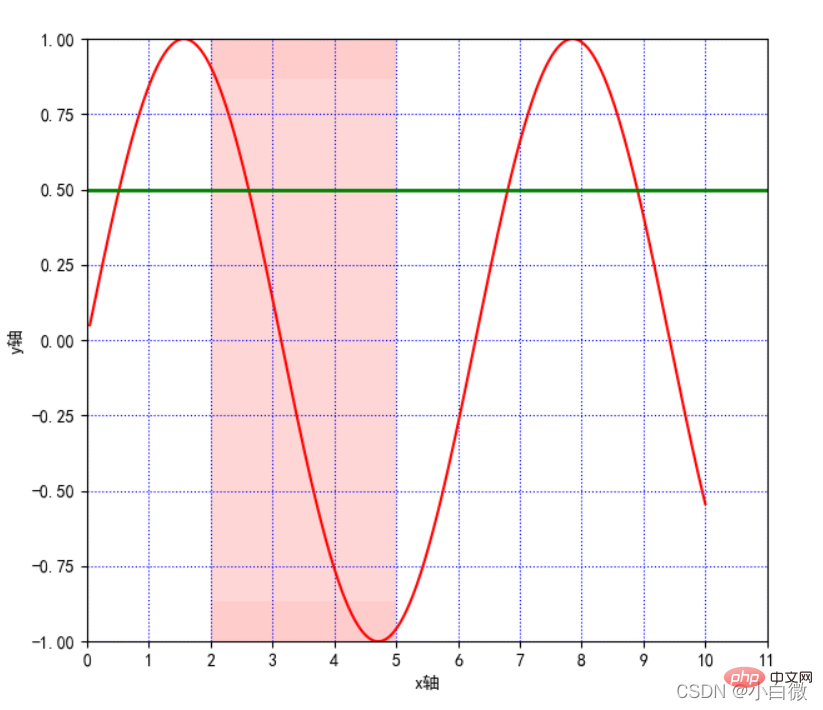# Python可视化总结之matplotlib.pyplot基本参数详解【相关推荐：Python3视频教程

## 1.matplotlib简介

matplotlib 库是 Python 中绘制二维和三维图表的数据可视化工具

pyplot：面向当前图

axes：面向对象

Pylab：沿用 matlab 风格

```import matplotlib.pyplot as plt
import numpy as np```

## plot()：展示变量的趋势变化

x，y：x，y 轴上的数值

c：设置颜色

ls：折线图的线条风格

lw：折线图的线条宽度

label：标记图形内容的标签文本

alpha：透明度

**kwargs：指定使用的是 line2D 属性

## 2.1. figure()：背景颜色

edgecolor=None, frameon=True, FigureClass=Figure, clear=False, **kwargs)

num ：

figsize：以英寸为单位的宽高，缺省值为 rc figure.figsize (1 英寸等于 2.54 厘米)

dpi：图形分辨率，缺省值为 rc figure.dpi

facecolor：背景色

```plt.figure(figsize=(10, 10))
x = np.linspace(0.05, 10, 1000)  # 在0.05到10的区间中，等差选取1000个，端点不属于
y = np.sin(x)
plt.rcParams['font.sans-serif'] = ['SimHei']
plt.rcParams['axes.unicode_minus'] = False
plt.plot(x, y,
color='red',
ls='-',
label='sinx')
plt.show()```

xmin：x 轴上的最小值

xmax：x 轴上的最大值

## 2.3 xlabel()和 ylabel()：设置 x，y 轴的标签文本

fontsize：数字或者（small，large，medium）

verticalalignment：距离坐标轴的位置（top，bottom，center，baseline）

hoizontalalignment：位置（center，right，left）

ratation：位置（vertical，horizontal，vertical）

bbox：添加边框

## 2.5 axhline()：绘制平行于 x 轴额度水平参考线

y：水平参考线的出发点

```plt.figure(figsize=(10, 10))
x = np.linspace(0.05, 10, 1000)  # 在0.05到10的区间中，等差选取1000个，端点不属于
y = np.sin(x)
plt.rcParams['font.sans-serif'] = ['SimHei']
plt.rcParams['axes.unicode_minus'] = False
plt.plot(x, y,
color='red',
ls='-',
label='sinx')
plt.xlim(1, 10)
plt.ylim(-1, 1)
plt.xlabel('x轴')
plt.ylabel('y轴')
plt.grid(ls=':',
color='blue')  # 设置网格，颜色为蓝色
plt.axhline(0.5, color='green', lw=2, label="分割线")  # 绘制平行于x轴的水平参考线，绿色，名称
plt.show()```（上图中绿色的线即为axjline（）添加的参考线）

## 2.6 axvspan()：绘制垂直于 x 轴的参考区域

xmin：参考区域的起始位置

xmax：参考区域的终止位置

facecolor：参考区域的填充颜色

alpha：参考区域填充颜色的透明度,[0~1]

```plt.axvspan(xmin=2,
xmax=5,
facecolor='r',
alpha=0.2)  # 绘制垂直于x轴的参考区域```

## 2.7 xticks(),yticks()

`plt.xticks(list(range(0, 12, 1)))  # 调整刻度范围和刻度标签`## 2.8 annotate()：添加图形内容细节的指向型注释文本

s：注释文本内容

xy：被注释的坐标点

xytext：注释文字的坐标位置

weight：设置字体线形(Ultralight，light，normal，regular，book，medium，roman，semibold，demibold，demi,bold，heavy，extrabold，black）

color：设置字体颜色；也可以设置 RGB 或 RGBA 类型的颜色；但必须为[0,1]之间的浮点 数

xycoords= 参数如下

figure points：图左下角的点

figure pixels：图左下角的像素

figure fraction：图的左下部分

axes points：坐标轴左下的点

axes pixels：坐标轴左下的像素

data：使用被注释对象的坐标系统

arrowprops：箭头参数，参数类型为字典 dict

width：箭头的宽度

shrink：总长度的一部分，从两端“收缩”

facecolor：箭头颜色（如果设置了 arrowstyle 关键字，上面的参数都不可以用，可

-

->

-[

|-|

-|>

<->

<|-

<|-|>

fancy

simple

wedge

```plt.annotate('local',
xy=(2, 1),
xytext=(0.5, 0.5),
weight='bold',
color='red',
xycoords="data",
arrowprops=
dict(arrowstyle="->", connectionstyle='arc3', color='b'),
bbox=
dict(boxstyle="rarrow",
fc="yellow",
ec='k',
lw=1,
alpha=0.5)
)```## 2.9 bbox：给标题增加外框

（boxstyle：方框外形；circle：椭圆；darrow：双向箭头；larrow：箭头向左；rarrow：箭

## 2.10 . text()：添加图形内容细节的无指向型注释文本(水印）

x,y：表示坐标轴上的值

weight：

ultralightlight

normal

regular

book

medium

roman

semibold

demibold

demi

bold

heavy

extrabold

black

xycoodrds：

figure points：图左下角的点

figure pixels：图左下角的像素

figure fraction：图的左下部分

axes points：坐标轴左下的点

data：使用被注释对象的坐标系统

arrowprops：箭头参数，参数类型为字典 dict

width：箭头的宽度

shrink：总长度的一部分，从两端“收缩”

facecolor：箭头颜色

bbox：给标题增加外框

boxstyle：方框外形

circle：椭圆

darrow：双向箭头

larrow：箭头向左

rarrow：箭头向右

round：圆角矩形

round4：椭长方形

roundtooth：波浪形边框 1

sawtooth：波浪形边框 2

square：长方形

```plt.text(1, 1,
"y=sinx",
weight='bold',
color ='b')```

## 2.11. title()：添加图形内容的标题

`plt.title("正弦函数")`## 2.12. legend()：标示不同图形的文本标签图例

best

upper right

upper left

lower left

lower right

right

center left

center right

lower center

upper center

center

`plt.legend(loc="lower left")  # 设置图例位置`## 2.13 table()：向子图中添加表格

plt.table(cellText=None, cellColours=None, cellloc='right' ,colWidths=None,

rowLabels=None, rowColours=None, collLabels=None, colColours=None,

collloc='center', loc='bpttpm', bbox=None, edges='closed', **kwargs)

cellText：表格单元格文本。类型为二维字符串列表

cellColours：表格单元格背景色。类型为二位颜色值列表

cellloc：表格单元格文本的对齐方式。默认值为right

colWidths：表格单元格宽度。类型为浮点数列表

rowLabels：表格行表头文本。类型为字符串列表

rowColours：表格行表头背景色。类型为颜色列表

colLabels：表格列表头文本。类型为字符串列表

colLoc：表格行表头文本对齐方式。默认 right

colColours：表格列表头背景色。类型为颜色列表

loc：单元格相对于子图的位置

bbox：绘制表格的边界框，如果此参数不为 None，将会覆盖 loc 参数

edges：单元格边线，该属性会影响各类单元格背景色

## 3. 完整代码显示

```import matplotlib.pyplot as plt
import numpy as np

plt.figure(figsize=(10, 10))
x = np.linspace(0.05, 10, 1000)  # 在0.05到10的区间中，等差选取1000个，端点不属于
y = np.sin(x)
plt.rcParams['font.sans-serif'] = ['SimHei']
plt.rcParams['axes.unicode_minus'] = False
plt.plot(x, y,
color='red',
ls='-',
label='sinx')
plt.xlim(1, 10)
plt.ylim(-1, 1)
plt.xlabel('x轴')
plt.ylabel('y轴')
plt.grid(ls=':',
color='blue')  # 设置网格，颜色为蓝色
plt.axhline(0.5, color='green', lw=2, label="分割线")  # 绘制平行于x轴的水平参考线，绿色，名称
plt.axvspan(xmin=2,
xmax=5,
facecolor='r',
alpha=0.2)  # 绘制垂直于x轴的参考区域
plt.axhspan(ymin=(-3**0.5)/2,
ymax=(3**0.5)/2,
facecolor='w',
alpha=0.2)

plt.legend(loc="lower left")  # 设置图例位置
plt.annotate('local',
xy=(2, 1),
xytext=(0.5, 0.5),
weight='bold',
color='red',
xycoords="data",
arrowprops=
dict(arrowstyle="->", connectionstyle='arc3', color='b'),
bbox=
dict(boxstyle="rarrow",
fc="yellow",
ec='k',
lw=1,
alpha=0.5)
)
plt.xticks(list(range(0, 12, 1)))  # 调整刻度范围和刻度标签
plt.text(1, 1,
"y=sinx",
weight='bold',
color ='b')
plt.title("正弦函数")
plt.show()```

```plt.rcParams['font.sans-serif'] = ['SimHei']
plt.rcParams['axes.unicode_minus'] = False```

## 4.折线图的线条风格

```   -：实线样式
--：短横线样式
-.：点划线样式
：：虚线样式
.：点标记
O：圆标记
V：倒三角标记
^：正三角标记
<：左三角标记
>：右三角表示
1：下箭头标记13
2：上箭头标记
3：左箭头标记
4：右箭头标记
S：正方形标记
p：五边形标记
*：星形标记
H：六边形标记
+：加号标记
X：x 标记
D：菱形标记
|：竖直线标记
_：水平线标记```

## 5. 常用颜色缩写

```b 蓝色
g 绿色
r 红色
c 青色
m 品红色·
y 黄色
k 黑色
w 白色```

【相关推荐：Python3视频教程• 相关标签：python
• 相关文章

相关视频